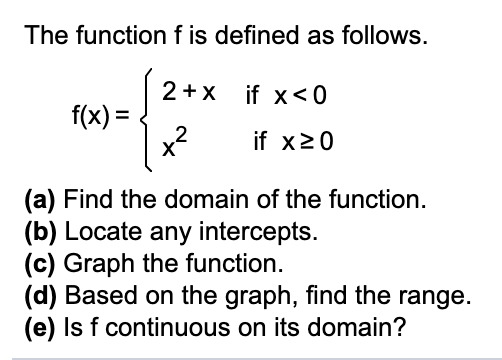Question
6 viewshelp_outlineImage TranscriptioncloseThe function f is defined as follows. 2+x if x<0 f(x) = 2 x? %3D if x20 (a) Find the domain of the function. (b) Locate any intercepts. (c) Graph the function. (d) Based on the graph, find the range. (e) Is f continuous on its domain? fullscreen
check_circle

Step 1

Given function is

Step 2

The function f(x) is defined everywhere on real line.

Therefore, the domain of the function is set of real numbers.

Step 3

For the y intercept set x = 0

At x = 0 we have f(x) = x2, so f(0) = 0.

Thus, the y intercept is (0, 0)

The point at which  f(x) = 0 is called x intercept.

The given functio...

### Want to see the full answer?

See Solution

#### Want to see this answer and more?

Solutions are written by subject experts who are available 24/7. Questions are typically answered within 1 hour.*

See Solution
*Response times may vary by subject and question.
Tagged in

### Other